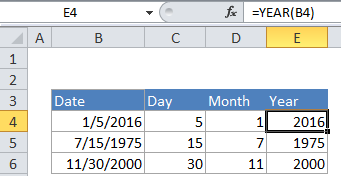# Get work hours between dates in Excel

To calculate the total number of work hours between two dates, you can use a formula based on the NETWORKDAYS function, where “start” is the start date, “end” is the end date, “holidays” is a range that includes dates, and “hours” is the number of work hours in a workday.

## Formula

`=NETWORKDAYS(start,end,holidays)*hours`## Explanation

In the example shown, the formula in D7 is:

`=NETWORKDAYS(B7,C7,holidays)*8`

where “holidays” is the named range G6:G8.

### How this formula works

This formula uses the NETWORKDAYS function calculate total working days between two dates, taking into account weekends and (optionally) holidays. Holidays, if provided, must be a range  of valid Excel dates. Once total work days are known, they are simply multiplied by a fixed number of hours per day, 8 in the example shown.

Also See:   How to calculate workdays per month in Excel

The NETWORKDAYS function includes both the start and end date in the calculation, and excludes both Saturday and Sunday by default. The function will also exclude holidays when then are provided as the “holidays” argument as a range of valid dates.

In these example shown, the first two formulas use the NETWORKDAYS function.

```D6=NETWORKDAYS(B6,C6)*8 // no holidays
D7=NETWORKDAYS(B7,C7,holidays)*8 // holidays provided```

If your workweek includes days other than Monday through Friday, you can switch to the NETWORKDAYS.INTL function, which provides a “weekend” argument that can be used to define which days of the week are workdays and weekend days. NETWORKDAYS.INTL can be configured just like NETWORKDAYS, but it provides an additional argument called “weekend” to control which days in a week are considered workdays.

Also See:   How to calculate working days left in month in Excel

The next 4 formulas use the NETWORKDAYS.INTL function:

```D8=NETWORKDAYS.INTL(B8,C8)*8 // Mon-Fri, no holidays
D9=NETWORKDAYS.INTL(B9,C9,11)*8 // Mon-Sat, no holidays
D10=NETWORKDAYS.INTL(B10,C10)*8 // M-F, no holidays
D11=NETWORKDAYS.INTL(B11,C11,1,holidays)*8 // M-F, w/ holidays```

Click the function names above to learn more about configuration options.

### Custom work schedule

This formula assumes all working days have the same number of work hours. If you need to calculate work hours with a custom schedule where work hours vary according to the day of week, you can try a formula like this:

`=SUMPRODUCT(MID(schedule,WEEKDAY(ROW(INDIRECT(start&":"&end))),1)*ISNA(MATCH(ROW(INDIRECT(start&":"&end)),holidays,0)))`